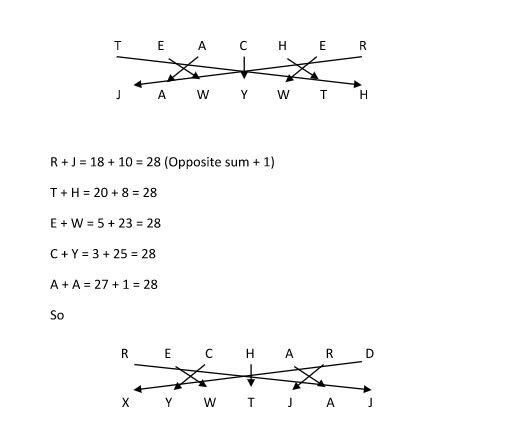Examdays team consistently providing study material on SSC CHSL 2018 SSC CGL and Bank exams, today we’re discussing Coding and Decoding concepts, where a candidate can score more marks with easy and simple methods. Understand the concept and practice more and more examples and take mock tests online before the final examination.

What is Coding and Decoding Concept? and How it implemented on Exam.?

Coding is nothing but encrypting normal information to secure information under a special type of codes are used, these codes should understand between the transmitter and receiver only but not any third party person. Which is also called as a confidential information.

Coding: Converting low-level information to high-level information

Decoding: Converting high-level information to low-level information.

Before starting live examples, please follow the below-specified tips and tricks to solve coding and decoding problems.

• Take a piece of paper and write English Aplphabetics from A, B, C, D, E…… till Z and code them with numbers like A=1, B=2, C=3, D=4…….soon z=26.
• Using above scenario code them in reverse order like A=26, B=25, C=24…..Soon Y=2, Z=1. (Later you understand the concept, why you wrote in reverse order too)
• Remember, the pair of English letters or Pair of numerical numbers also plays a vital role in solving coding and decoding concepts.

Let proceed with the type of examples, so you can understand the more easily.

Examples#1:

If in a certain language, EXAMDAYS is coded as FYBNEBZT, how is HOLIDAYS coded in that code?

Solution:

The given code is EXAMDAYS and it written in Decode format is FYBNEBZT.

Observe the above decode code carefully, which is next series of English Alphabetic A, B, C, D…..X, Y, Z.

E letter was written as F

X letter was written as Y

A letter was written as  B

M Letter was written as N

D Letter was written as E

Y Letter was written as Z

S letter was written as T

In the same way, you can proceed with next word “HOLIDAYS”….. The solution for it is >>>IPMJEBZT.

Example#2

Solve the problem using example#1 concept.

If in a code language, HARRY is written as IBSSZ and LAPPY is written as MBQQZ, how will NETMARKET be written in that code?

Example#3:Example#4

In a certain code language,

‘296’ means ‘examdays and love’;

‘324’ means ‘see examdays show’  and

‘451’ means ‘show are agree‘.

Which of the following digits stands for ‘see’?

Solution

The above is tricky to solve, each number is attached with one specified word, where we can arrange the exact order by removing common terms.

both 296 & 324 is the common number is 2 and in words, the common term is examdays… so that, 2 belongs to examdays word.

both 324 and 451  is the common number is 4 and in words, the common term is show… so that, 4 belongs to show the word.

The remaining answer is followed by the same number under the same group words.

Post the questions for solutions in below comment box or you can send it to [email protected]